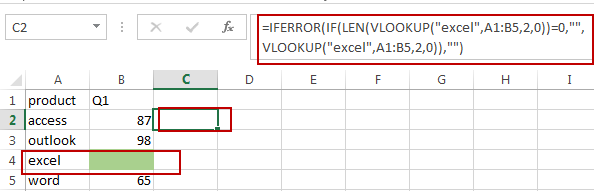# How to Vlookup to Return Blank Instead of 0 or NA Error in Excel

This post will guide you how to vlookup and return blank instead of 0 or #N/A error in Excel. How do I vlookup returning blank instead of 0 or #N/A when no data matched in Excel.

## Vlookup to Return Blank Instead of 0 or #N/A

When you use vlookup function to search a value, and it will return the searched value, and if the matched cell is blank, it will return 0. Or if the matched cell is not found, it will return #N/A error.  If you do not want to return blank instead of 0 or #N/A for those two cases. How to use the VLOOKUP function to create a formula in Excel.

Assuming that you have a list of data in Range of Cells A1:B5, and you want to find “excel” text string in range A1:B5, and return the corresponding value in column B.

If you want to return blank instead of 0 or #N/A Error, you can use a formula based on the IFERROR function, the IF function, the LEN function and the VLOOKUP function. Like this:

`=IFERROR(IF(LEN(VLOOKUP("excel",A1:B5,2,0))=0,"",VLOOKUP("excel",A1:B5,2,0)), "")`

Type this formula into a blank cell, and press Enter key in your keyboard.Let’s see how this formula works:

The first VLOOKUP formula will lookup value “excel” in range A1:B5, if TRUE, then return the corresponding value in Column B. and then the returned value passed into LEN function as it argument.

The Len function will return the length of the returned value by VLOOKUP Function. If the length is equal to 0, it indicates that the matching value is blank.

The IF formula will check if the length value is 0 or not, if it is equal to 0, then return blank value, otherwise, return the matched value.

The IFERROR function will check if the returned value by IF is #N/A, if true, return blank value.

### Related Functions

• Excel IF function
The Excel IF function perform a logical test to return one value if the condition is TRUE and return another value if the condition is FALSE. The IF function is a build-in function in Microsoft Excel and it is categorized as a Logical Function.The syntax of the IF function is as below:= IF (condition, [true_value], [false_value])….
• Excel VLOOKUP function
The Excel VLOOKUP function lookup a value in the first column of the table and return the value in the same row based on index_num position.The syntax of the VLOOKUP function is as below:= VLOOKUP (lookup_value, table_array, column_index_num,[range_lookup])….
• Excel IFERROR function
The Excel IFERROR function returns an alternate value you specify if a formula results in an error, or returns the result of the formula.The syntax of the IFERROR function is as below:= IFERROR (value, value_if_error)….
• Excel LEN function
The Excel LEN function returns the length of a text string (the number of characters in a text string).The syntax of the LEN function is as below:= LEN(text)…